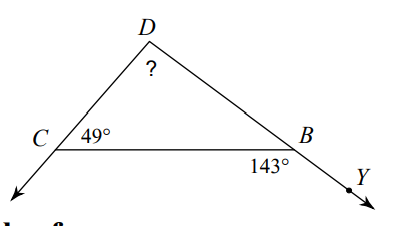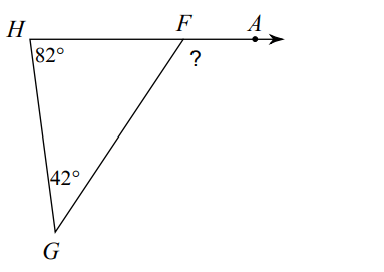Triangle Inequality Theorem
Triangle Sum Theorem
Vocabulary
Exterior Angle Theorem
Random
100

Can these three sides make a triangle?

6, 7, 9

Yes

100

What is the third angle of the triangle?

50, 45, B

85

100

A straight angle is __________ degrees.

180

100

If your remote interior angles are 24 degrees and 70 degrees, what is the measure of the exterior angle?

94 degrees

100

Can you add two fractions if they don't have the same denominator?

No, you have to change it to the same denominator before adding.

200

Can these three sides make a triangle?

10, 8, 19

No

200

What is the third angle of the triangle?

32, 63, B

85

200

Complementary angles add up to _______ degrees.

90

200

If the one of the remote interior angles is 75 degrees and the exterior angle is 100 degrees, how many degrees is the unknown remote interior angle?

25 degrees

200

_______ is the opposite operation of multiplying.

Dividing

300

Can these three sides make a triangle?

13, 4, 9

No

300

What is the third angle of the triangle?

15, 86, X

79

300

Supplementary angles add up to _______ degrees.

180

30094 degrees

300

An exponent tells us how many time to _________ a base.

Multiply
400

What could the third side of the triangle be?

7, 5, ?

11 or less

400

Use the three angles to solve for x.

25, 140, 3x+3

x = 4

400

What is a Theorem?

A rule.

40060 degrees

400

Solve for x

3x +15= 15

x= 0

500

What could the third side of the triangle be?

4, 5, ?

8 or less

500

Use the three angles to solve for x.

35, 90, 5x + 10

9

500

Two angles that add up to 180 degrees are called _________________ angles.

Supplementary

500124 degrees

500
Combine like terms

2x + 5y + x - 4y + 81

3x + y + 81

Click to zoom